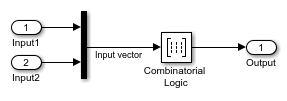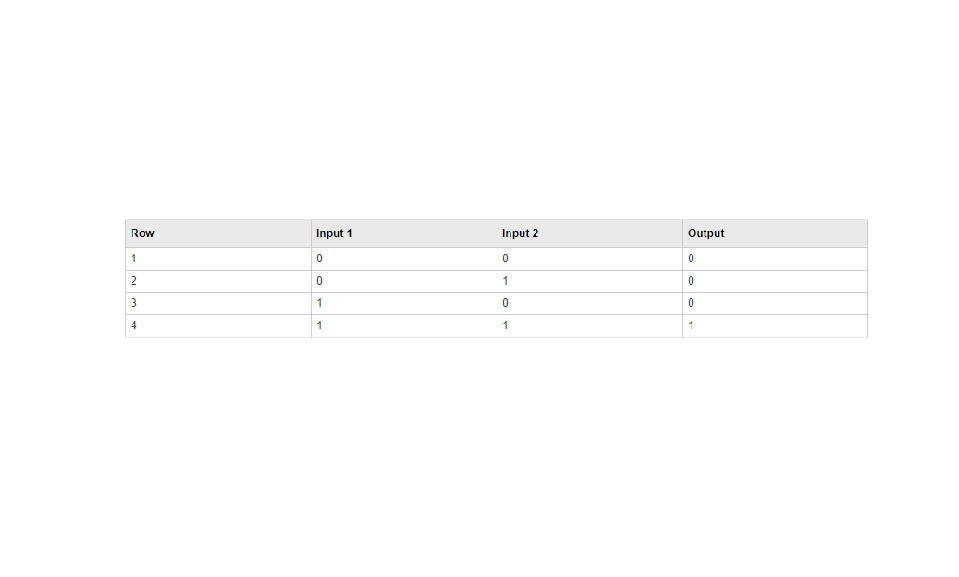The following table indicates the combination of inputs that generate each output. The input signal labeled `Input` corresponds to the column in the table labeled Input 1. Similarly, the input signal `Input 2` corresponds to the column with the same name. The combination of these values determines the row of the Output column of the table that is passed as block output. For example, if the input vector is [1 0], the input references the third row: